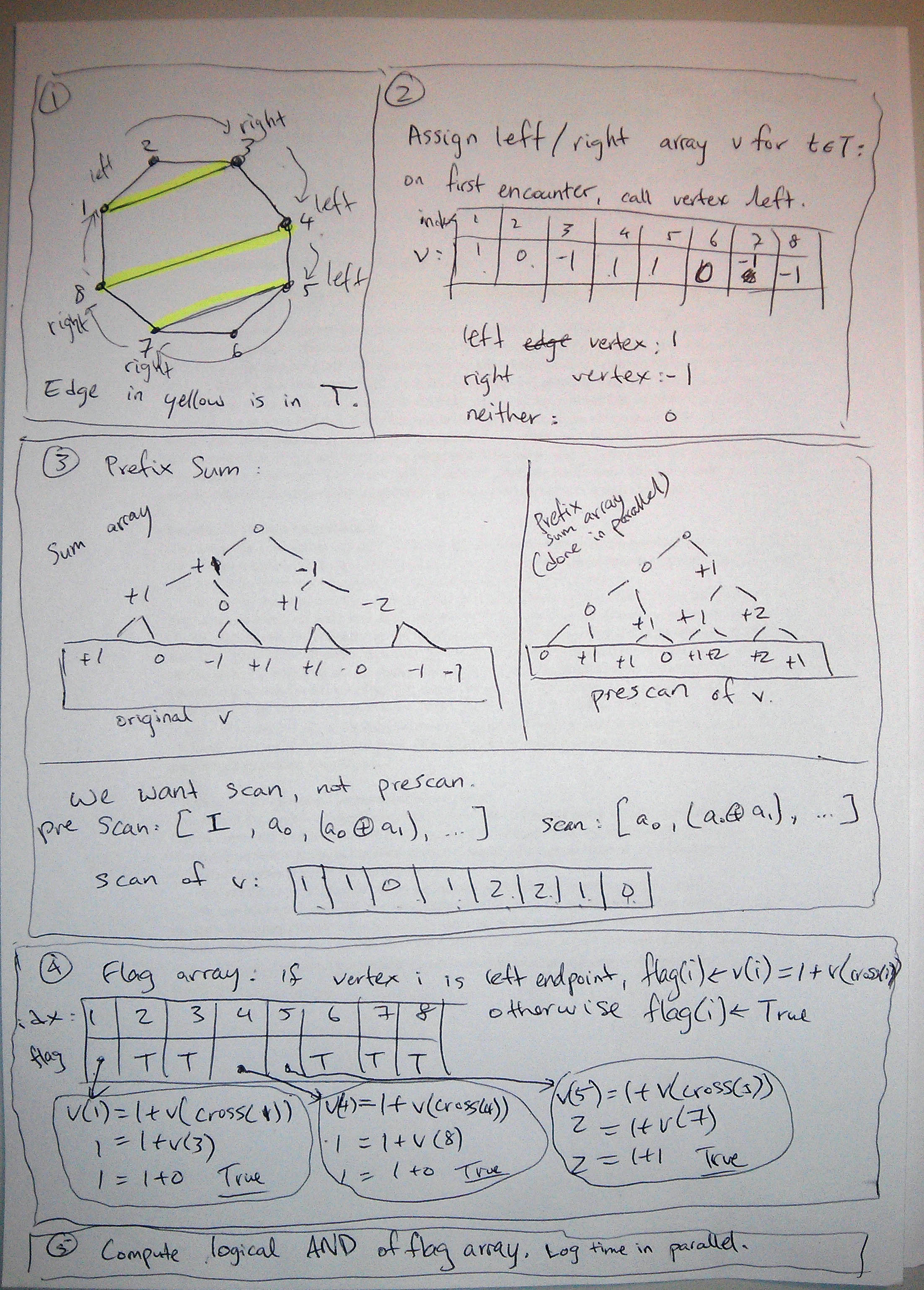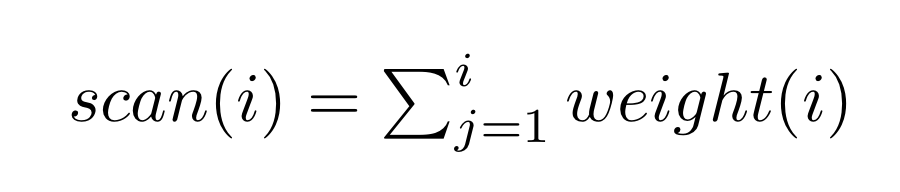# Parallel Algorithms: Prefix Sum Application On Graph

Preparing for advanced algorithms exam (CS450 at EPFL), and I just truly understood a problem. First reaction is: “TIL prefix sum is a stack. So powerful!”.

The problem:

You are given a cycle of n vertices with an additional set T of edges between these n vertices (in addition to the n edges that form the cycle). You are to determine whether it is possible to draw the edges of T inside the cycle in such a manner that no two edges cross. Develop a parallel algorithm that runs on O(logn) time and uses linear total work to solve this problem.

The given solution, by Professor Bernard Moret:

The key point to note is that the state of stack (and thus of the entire computation) when the left endpoint is encountered must equal the state of the stack when the matching right endpoint has been processed—and this must hold for every edge. This is a property that we can verify by a simple prefix sums operation: we assign to each left endpoint a value of +1 and to each right endpoint a value of -1; this step can be done in constant parallel time. Then we compute the prefix sum of these values. Finally, we check the matching: the prefix sum value of a left endpoint must equal one plus the prefix sum value of the matching right endpoint. These checks can be carried out in constant parallel time, yielding a Boolean value at each vertex; these values are then logically ANDed together in logarithmic time and linear work with the standard binary tree of operations.

Thus our algorithm uses the following steps:

```1. If necessary, rank the cycle.

2. If vertex i is a left endpoint, set v(i) ← 1;
if vertex i is a right endpoint, set v(i) ← −1;
otherwise set v(i) ← 0.

3. Run prefix sums on the array v( ). So v( ) becomes a scan (not a prescan).

4. If vertex i is a left endpoint,
set flag(i)←v(i)=1+v(cross(i));
otherwise set flag(i) ← true.

5. Compute the logical AND of the flag( ) array.
```

The algorithm runs in logarithmic time and linear work (assuming we take advantage of accelerated cascading).

This is so powerful. The solution is hard to understand without some visualization. I followed the steps and have an example:Note the scan version of prefix sum can be computed sequentially as:For the ith vertex. E.g.
1 = 1
1 = 1 + 0
0 = 1 + 0 – 1
1 = 1 + 0 – 1 + 1
2 = 1 + 0 – 1 + 1 + 1
2 = 1 + 0 – 1 + 1 + 1 + 0
1 = 1 + 0 – 1 + 1 + 1 + 0 – 1
0 = 1 + 0 – 1 + 1 + 1 + 0 – 1 – 1

And there you have it!